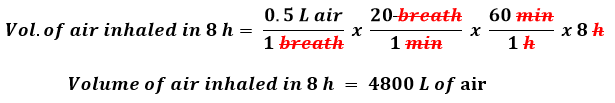# Problem: Section 1.7 showed that in 1997 Los Angeles County air had carbon monoxide (CO) levels of 15.0 ppm. An average human inhales about 0.50 L of air per breath and takes about 20 breaths per minute.How many milligrams of carbon monoxide does the average person inhale in an 8 hour period for this level of carbon monoxide pollution? Assume that the carbon monoxide has a density of 1.2 g/L. (Hint: 15.0 ppm CO means 15.0 L per 106 air.)

###### FREE Expert Solution

The following steps have to be taken to determine the mass (in milligrams) of CO inhaled:

Step 1: Finding the Volume of air inhaled in 8 hours.

The Volume of CO inhaled per breath = 0.5 L

We can use the following conversion to find the volume of air inhaled in 8 h.

Recall that, 1 hour = 60 minThe volume of air inhaled in 8 hours by an average human is 4800 L.

80% (180 ratings)###### Problem Details

Section 1.7 showed that in 1997 Los Angeles County air had carbon monoxide (CO) levels of 15.0 ppm. An average human inhales about 0.50 L of air per breath and takes about 20 breaths per minute.

How many milligrams of carbon monoxide does the average person inhale in an 8 hour period for this level of carbon monoxide pollution? Assume that the carbon monoxide has a density of 1.2 g/L. (Hint: 15.0 ppm CO means 15.0 L per 106 air.)

Frequently Asked Questions

What scientific concept do you need to know in order to solve this problem?

Our tutors have indicated that to solve this problem you will need to apply the Density concept. You can view video lessons to learn Density. Or if you need more Density practice, you can also practice Density practice problems.

What professor is this problem relevant for?

Based on our data, we think this problem is relevant for Professor Mohanty's class at OKSTATE.